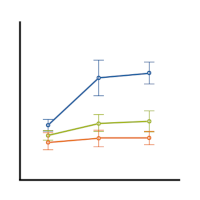# 3 Situations When it Makes Sense to Categorize a Continuous Predictor in a Regression ModelIn many research fields, a common practice is to categorize continuous predictor variables so they work in an ANOVA. This is often done with median splits. This is a way of splitting the sample into two categories: the “high” values above the median and the “low” values below the median.

### Reasons Not to Categorize a Continuous Predictor

There are many reasons why this isn’t such a good idea:

• The median varies from sample to sample. So the categories in different samples have different meanings.
• All values on one side of the median are considered equivalent. This means any variation within the category is ignored, and two values right next to each other on either side of the median are considered different.
• You’re losing information about the real relationship between the predictor variable and the outcome.
• The categorization is completely arbitrary. A ‘High” score isn’t necessarily high. If the scale is skewed, as many are, even a value near the low end can end up in the “high” category.

### Exceptions When it Works

But it can be very useful and legitimate to choose whether to treat a numeric independent variable as categorical or continuous. Knowing when it is appropriate and understanding how it affects interpretation of regression coefficients allows the data analyst to find real relationships that might otherwise have been missed.

The main thing to understand is that the general linear model doesn’t really care if your predictor is continuous or categorical. But you, as the analyst, choose the information you want from the analysis based on the coding of the predictor. Numerical predictors are usually coded with the actual numerical values. Categorical variables are often coded with dummy variables—0 or 1.

Without getting into the details of coding schemes, when all the values of the predictor are 0 and 1, there is no real information about the distance between them. There’s only one fixed distance—the difference between 0 and 1. So the model returns an estimate of the regression coefficient that gives information only about the response—the difference in the means of the response for the two groups.

But when a predictor is numeric, the regression coefficient uses the information about the distances between the predictor values—the slope of a line. The slope uses both the horizontal distances—the variation in the predictor—and the vertical distances—the variation in the response.

So when deciding whether to treat a predictor as continuous or categorical, what you are really deciding is how important it is to include the detailed information about the variation of values in the predictor.

As I already mentioned, median splits create arbitrary groupings that eliminate all detail. Some other situations also throw away the detail, but in a way that is less arbitrary and that may give you all the information you need:

### 1. Sometimes the quantitative scale reflects meaningful qualitative differences.

For example, a well tested depression scale may have meaningful cut points that reflect “non”, “mild”, and “clinical” depression levels. Likewise, some quantitative variables have natural meaningful qualitative cut points.

One example would be age in a study of retirement planning. People who have reached retirement age and eligibility for pensions, Social Security and Medicare, will have qualitatively different situations than those who have not. So the difference between ages 64 and 65 isn’t just one year in this context. It’s a change in circumstances.

### 2. When there are few values of the quantitative variable, fitting a line may not be appropriate.

Mathematically, only two values are necessary to fit a line. But it won’t be a good regression line. The line will be forced through both points, reflecting the means exactly.

So if that retirement study is comparing workers at age 35 to those at age 55, the line will be unlikely to be a true representation of the real relationship. Comparing the means of these qualitatively different groups would be a better approach. Having 3 points is better than 2, but the more, the better. If the study included all ages between 35 and 55, fitting a line would actually give more information.

### 3. When the relationship is not linear.

As few as 5 or 6 values of the predictor can be considered continuous if they follow a line. But what if they don’t? You can learn a lot more about the relationship between your variables by looking at which means are higher than which others, rather than trying to fit a complicated non-linear model.

In the same example about retirement planning, say you have data for workers aged 30, 35, 40, 45, 50, and 55. If the first four ages have similar mean amounts of retirement planning, but at age 50 it starts to go up, and at 55 it jumps much higher, one approach would be to fit some sort of exponential model or step function. Another approach would be to consider age as categorical, and see at which age retirement planning increased.

The key thing here is we’re treating each distinct value of age as its own category. We can only do it because in this study, age was measured at discrete values, not continuously.

So, believe it or not, sometimes it’s a good idea to categorize a continuous predictor variable. While you don’t want to categorize a variable just to get it to fit into the analysis you’re most comfortable with, you do want to do it if it will help you understand the real relationship between your variables.Four Critical Steps in Building Linear Regression Models
While you’re worrying about which predictors to enter, you might be missing issues that have a big impact your analysis. This training will help you achieve more accurate results and a less-frustrating model building experience.

### Related Posts

1.Matthew Healy says